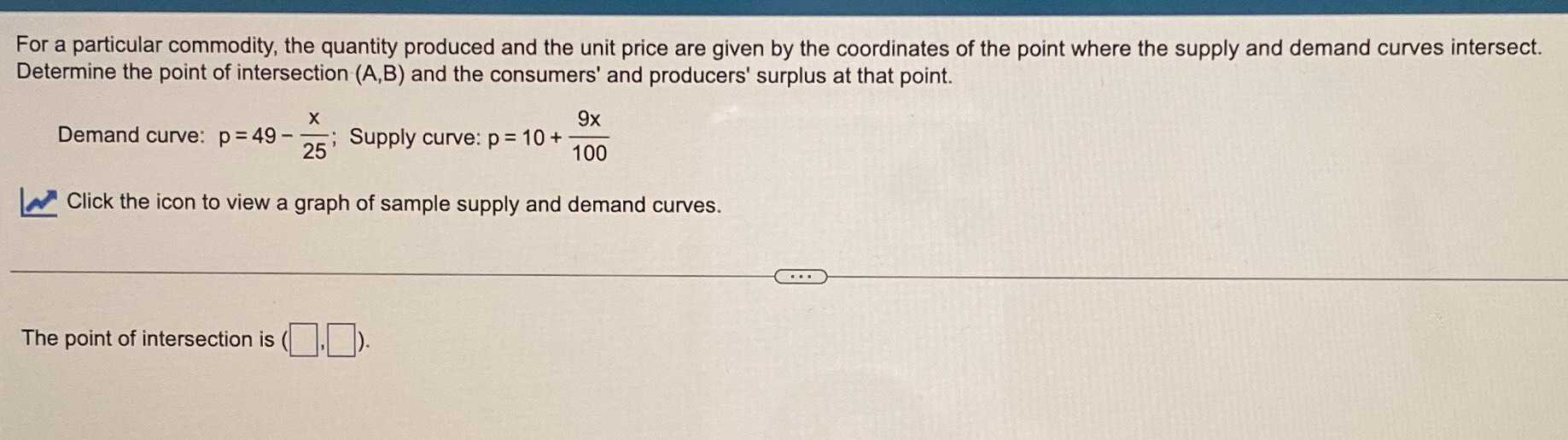### Still have math questions?

Algebra
QuestionFor a particular commodity, the quantity produced and the unit price are given by the coordinates of the point where the supply and demand curves intersect. Determine the point of intersection $$( A , B )$$ and the consumers' and producers' surplus at that point. Demand curve: $$p = 49 - \frac { x } { 25 }$$ ; Supply curve: $$p = 10 + \frac { 9 x } { 100 }$$

Click the icon to view a graph of sample supply and demand curves. The point of intersection is $$\square$$.